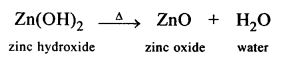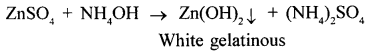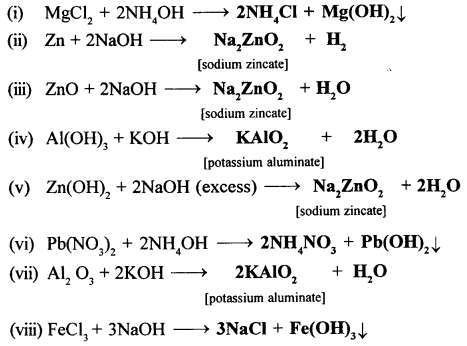# New Simplified Chemistry Class 10 ICSE Solutions – Analytical Chemistry

## New Simplified Chemistry Class 10 ICSE Solutions – Analytical Chemistry – Use of Ammonium And Sodium Hydroxide

Viraf J Dalal Chemistry Class 10 Solutions and Answers

2003

QUESTIONS

Question 1.
Write the observations and balanced equations for the following reactions:

1. Sodium hydroxide is added drop-wise till in excess to a solution of zinc sulphate.
2. Ammonium hydroxide is added in excess to a solution of copper sulphate.

(1) A white ppt formed redissolves in excess of sodium hydroxide solution.(2) Pale blue ppt formed dissolves in excess of ammonium hydroxide solution to form deep blue solution.
CuSo4 + 2NH4H → (NH4)2SO4 + Cu(OH)22004

Question 1.
Sodium hydroxide solution is added first in a small amount then in excess to the aqueous salt solutions of
(a) copper [II] sulphate,
(b) zinc nitrate,
(d) iron
State in each case:

1. the colour of the precipitate when NaOH is added in a small quantity;
2. the nature of the precipitate (i.e. soluble or insoluble) when NaOH is added in excess.

(1)
(a) Blue
(b) White
(c) White
(d) Reddish brown

(2)
(a) Insoluble
(b) Soluble
(c) Soluble
(d) Insoluble.

Question 2.
Write balanced equations for –
(a) Aluminium
(b) Zinc – is warmed with NaOH (caustic soda)
(a) Al + 2NaOH + 2H2o → 2NaAlo2 + 3H2
(b) Zn + 2NaOH → Na2Zno2 + H2

2005

Question 1.
The questions below refers to the following salt solutions listed A to F:
A: Copper nitrate, B: Iron [U] sulphate, C: Iron [III] chloride, D: Lead nitrate, E: Magnesium sulphate, F: Zinc chloride.

(1) Which soln. becomes a deep/inky blue colour when excess of ammonium hydroxide is added to it.
Ans. A: Copper nitrate

(2) Which solution gives a white precipitate with excess ammonium hydroxide solution.

2006

Question 1.
From the list of substances given – Ammonium sulphate,Lead carbonate, Chlorine, Copper nitrate ferrous sulphate- State a solution of the compound which gives a dirty green precipitate with sodium hydroxide.
Ferrous sulphate

Question 2.
Write a balanced equation for the reaction between – aluminium oxide and sodium hydroxide
Al2o3 + 2NaOH → 2NaAlo2 + H2O

Question 3.
Give one test to distinguish between the following – Iron (III) chlorine soln. and copper chloride solution.
Add NaOH solution in excess of the two solutions. The one in which white ppt. initially formed dissolves in excess of NaOH solution is Zn(No3)2 solution and the other is Ca(No3)2 solution.

2008

Question 1.
The salt which in soln. gives a pale green precipitate with NaOH solution and a white ppt. with BaCl2 soln. is:
(a) Iron (III) sulphate
(b) Iron (II) sulphate
(c) Iron (II) chloride
(d) Iron (III) chloride

2009

Question 1.
Find the odd one with reason (note: valency is not a criterion): Al(OH)3 Pb(OH)2 Mg(OH)2 Zn(OH)2
Mg(OH)2 [Because all others are amphoteric hydroxides]

Question 2.
Identity the substance P based on the information given below:
The deliquescent salt P, turns yellow on dissolving in water, and gives a reddish brown precipitate with sodium hydroxide solution.
Ferric chloride.

2010

Question 1.
Give an equation for –

1. ZnO reacts with NaOH solution.
2. Conversion of – Zn(No3)2 to Zn(OH)2

(1)(2)Question 2.

Select the correct answer from A, B, C –
A: Sodium hydroxide soln. B: A weak acid C: Dilute sulphuric acid. The solution which with zinc sulphate solution will give a white precipitate.
A: Sodium hydroxide soln.

2011

Question 1.
Choose the correct answer from the choices given:Hydroxide of this metal is soluble in NaOH solution. A: Magnesium
C: Silver
D: Copper

Question 2.
Sodium hydroxide solution is added to the solutions containing the ions mentioned in List 1.
List 1 –

(i) Pb2+ (ii) Fe2+ (iii)Zn2+ (iv) Fe 3+ (v) Cu2+ (vi)Ca2+
List 2 – Gives the details of the precipitate. A: Reddish brown B: White insoluble in excess, C: Dirty green D: White soluble in excess, E: White soluble in excess F: Blue.

Match the ions with their coloured precipitates in List 2.Question 3.
Give a balanced chemical equation in each case when-

1. Zinc oxide dissolves in sodium hydroxide.
Ans. ZnO + 2NaOH → Na2 Zno2 + H20
2. Zinc is heated with NaOH solution.
Ans. Zn + 2NaOH → Na2 Zno2 + H2

2012

Question 1.
Name: The gas evolved on reaction of Aluminium with boiling concentrated caustic alkali solution.
Ans.
Hydrogen gas

Question 2.
State one observation for:

1. Excess NH4OH soln. is added to Pb(No3)2
Ans. White ppt. insoluble in excess ammonium hydroxide.
2. NaOH soln. is added to FeCl3 in excess.
Ans. Reddish brown precipitate is formed which in insoluble in excess of NaOH.

2013

Question 1.
State two relevant observations for the reaction:
Ammonium hydroxide solution is added to copper (II) nitrate solution in small quantities and then in excess.
With small amount of ammonium hydroxide, a bluish white pre­cipitate is formed. This precipitate dissolves in excess of am­monium hydroxide to form a deep blue solution.

2014

Question 1.
State your observation: When excess sodium hydroxide is added to calcium nitrate solution.
White ppt. formed is spairingly soluble.2015

Question 1.
To a salt solution ‘Y’ a small quantity of ammonium hy­droxide solution is added slowly and then in excess. A pale blue precipitate is formed which dissolves in ex­cess to form a clear inky blue solution. Identify the posi­tive ion in the salt ‘Y’
Cu2+ (Copper ion)

2016

Question 1.
State your observations when ammonium hydroxide solution is added drop by drop and then in excess to each of the following solutions:
(1) copper sulphate solution
(2) zinc sulphate solution
(1)Pale blue precipitates are formed and then these precipitates dissolve in excess of ammonium hydroxide to form deep blue solution.
(2)When ammonium hydroxide is added drop by drop white gelatinous precipitate of zinc hydroxide is formed and on adding excess of ammonium hydroxide colourless solution is formed.

2017

Question 1.
State one relevant observation – Action of sodium hydroxide solution on iron [II] sulphate solution.
A dirty green precipitate is formed which is insoluble in excess of sodium hydroxide solution.

Question 2.
How will you distinguish between – Ammonium hydroxide & sodium hydroxide using CuS04
Sodium hydroxide forms a pale blue precipitate which is insoluble in excess of sodium hydroxide.
Ammonium hydroxide forms a pale blue precipitate which redissolves in excess of ammonium hydroxide to form deep blue colouration.

Question 1.
Salts of…………… (normal / transition) elements are generally coloured. From the ions K,+, Cr3+, Fe2+, Ca2+ So32-, Mno41-, No31- the ions generally coloured are
Normal; Cr3+, Fe2+, Mno41

Question 2.
The hydroxide which is soluble in excess of NaOH is [Zn(OH)2 / Fe(OH)3 / Fe(OH)2]
Zn(OH)2

Question 3.
The salt which will not react with NH4OH solution [ZnCl2/CuCl2/NH4 Cl/FeCl2]
NH4Cl

Question 4.
The substance/s which react/s with hot cone. NaOH soln. and undergoes a neutralization reaction…………….. [Al203/Al/Al(OH)3]
Al203

Question 5.
To distinguish soluble salts of zinc and lead, ……………….[NaOH/NH4OH] can be used.
NaOH

Question 6.
Oxides and hydroxide of certain metals i.e………… (iron/ zinc/copper/aluminium/magnesium/Iead] are amphoteric and react with……………[acids/alkali/acids and alkalis].
zinc, aluminium and lead; acids and alkalis
Complete the following equations:

1. MgCl2 + NH4OH→ ……… +………..
2. Zn + NaOH → ……… +…….
3. ZnO + NaOH → ……… +…………
4. Al(OH)3 + KOH → …….. +………..
5. Zn(OH)2 + NaOH (excess) → ………. + ………..
6. Pb(No3)2 + NH4OH → …… + ………..
7. Al2 O3 + KOH → ……. +…………
8. FeCl3 + NaOH → ………+ ……….